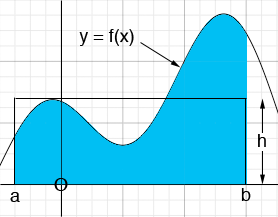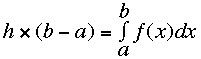SEARCH HOMEMath Central Quandaries & QueriesQuestion from vern, a student: Find the average rate of change of the function over the given interval. Compare this average rate of change with the instantaneous rates of change at the endpoints of the interval. f(X)=sinX for the interval [0,pi/6]?Hi Vern,

For a function y = f(x) whose graph is above the x-axis on the interval [a, b], the average value of the function on the interval from a to b is the height h so that the area under the graph of y = f(x) from a to b is the same as the area of the rectangle with base [a, b] and height h.That is the average of the function y = f(x) from a to b is the number h so thatIn your example yo want the average value of the rate of change of f(x) which is the derivative of f(x) so differentiate f(x) = sin(x) to obtain f '(x) = cos(x) and apply the definition of the average value to the function f '(x) = cos(x).

HarleyMath Central is supported by the University of Regina and The Pacific Institute for the Mathematical Sciences.